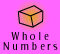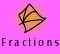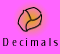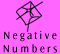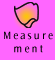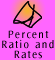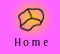| Meaning and Models | Key Ideas |
| Adding negative numbers | Subtracting negative numbers |Multiplying negative numbers | Dividing negative numbers |

 1. Write each of the following as directed numbers: a) 150 metres below sea level, -150 metres b) a fine of \$75, -\$75 c) a temperature drop of 8 degrees, -8 degrees d) 1973 metres above sea level, +1973 metres e) a lead of 25 metres, +25 metres f) a drop of 30 centimetres, -30 centimetres g) a loan of \$1500, -\$1500 h) a profit of \$300, +\$300 2. a) If the temperature in the city today was 11 degrees and it dropped by 5 degrees overnight, the minimum temperature was 11 - 5 = 6 degrees. b) If the temperature was only 8 degrees in The Dandenongs during the day and dropped by 10 degrees overnight, the minimum temperature reached was 8 - 10 = -2 degrees. c) The temperature at Mt Hotham last night was -3 degrees and has risen to 7 degrees today. The temperature rose from -3 to 7, that is the difference between 7 and (-3) which is found by subtraction: 7 - (-3) = 10. So although the temperature is only 7 degrees now is rose 10 degrees (from -3) overnight. 3. a) If Clara and Than actually climbed 100 metres and they started off at 20 metres below sea level then they climbed to '-20 + 100' metres above sea level, which is 80 metres above sea level. b) If Clara and Than climbed a further 450 metres, then they would now be 80 + 450 metres above sea level, i.e. 530 metres above sea level.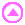1. a) 6 > -1 b) - 9 > -12 c) 0 > -3 d) 17 > -17 e) 6 > -5 > -22 f) -41 < 8 and -2 < 8 so -41<-2<8 2. Order the following sets of numbers from smallest to largest. a) -9, -7, -1, -1/3, 0, 0.5, 3, 7, 71 b) -15, -12, -9, -6, -3, 0, 0.15, 12 c) -157, -31, -4, -1.3, -0.4, 0, 1/5, 4 d) -419, -328, -325.36, -125, -2, 0, 12/15, 10, 12.5 3. a) 45 - 79 = -34 b) 53 - (-78) = 131 c) -34 + 9 = -25 d) (- 168) - 94 = -262 e) 67 - (- 5) + -2 = 70 f) -19 + (-5) - 17 - 3 = -44 4. Put the following numbers on a number line. 0.22, -1.8, 0.17, -0.17, 1/17, -1/17, 1, 1 1/4, 1/4. The numbers would be arranged in the following order on a number line: -2, -1.8, -1, -0.17, -1/17, 0, 1/17, 0.17, 0.22, 1/4, 1, 1 1/4, 2 5. Which is the greatest number? a) (-5), (-4) or (-1) Answer = -1 b) (-10) ,(0) or (+0.1) Answer = +0.1 c) (-0.1), (-0.2) or (-1/2) Answer = -0.11. a) 6 + (-3) = 3 b) -7 + 4 = -3 c) -8 + (-5) = -13 d) -12 + (-3) = -15 2. a) -14 + 6 = -8 b) -8 + 10 = 2 c) -22 + -13 = -35 d) -7 + (-14) = -21 e) -15 + 5 + (-8) + (-7) + 10 = -15 f) 13 + -9 + (-11) - 3 = -10 g) -22 + (-19) + 4 + (-6) + 2 = -41 h) -10 + (-11) + 1 + 2 - 3 = -21 3. The water level was at the full mark after Winter. After Summer it dropped by 5 metres and after Autumn it was raised by 1 metre. So, since Winter the water level has moved by, -5 + 1 metres (= -4 metres). This means it has dropped by 4 metres. Therefore, the current level of the reservoir is 4 metres below the full mark.1. a) 6 - (+3) = 3
b) -7 - 4 = -11
c) -8 - (-5) = -3
d) -8 + 5 = -3

2. a) -4 - 16 = -20
b) 10 - 19 = -9
c) -22 -13 = -35
d) -7 - (-14) = 7
e) -9 - (-5) + 15 - 7 - (-4) = 8
f) 12 - (-4) + (-3) - 9 + (-11) - 5 = -12
g) -6 + 8 - (-9) + 7 -(-10) = 28
h) -5 - (-17) - 25 + (-13) + 26 = 0

3.

The final balance is obtained by adding all the transactions.

 - 1235.99 583.00 299.00 - 25.75 - 60.00 784.21 201.00 545.47

4.

a) From level B2, you need to travel 1 level up to get to B1.

b) From level B2, you need to travel 2 levels up to get to ground level.

c) If I am shopping on level 5 of the shopping centre and I have parked on level B3, I need to travel 8 levels down to get to my car.

b If I park on level B2, I need to travel 4 levels up to get to level 2.

5.

Write each of the problems above as an addition or subtraction of negative numbers. The first is done for you.

a) (-1) - (-2) = 1 (the difference between B1 and B2)

or, (-2) + ? = 1, (-2) + (1) = -1

b) 0 - (-2) = 2 (the difference between ground and B2)

or, (-2) + ? = 0, (-2) + 2 = 0

c) (-3) - 5 = -8 (the difference between B3 and level 5)

or, 5 - ? = -3, 5 - (8) = -3

d) 2 - (-2) = 4 (the difference between level and B2)

or, (-2) + ? = 2, (-2) + 4 = 21. a) -3 x 2 = -6 b) 4 x -5 = -20 c) 7 x -1 = -7 d) -3 x 6 = -18 2. a) 2 x (4 x 5) -1 = 39 b) -17 x 4 x -3 = 204 c) -6 x (-5) + 15 + (-6) = 39 d) 3 - 4 + (5 x -8) = -41 3. Although there is no adequate model to show that -4 x -5 = 20 we can look at the patterns which emerge in the '4 times table' and the '-4 times table' or in the 'basic multiplication facts grid'. We can also use the distributive law to show that this must be the case. See Multiplying Directed Numbers for more detail. 4. (a) 14 x (3 - 7) + (8 x -3) = (14 x -4) + (-24) = -80 (b) (-4 - (-9)) x 2 + (-6) = (-4 + 9) x 2 + (-6) = (5 x 2) - 6 = 41. a) -16 ÷ -4 = 4 b) -40 ÷ 8 = -5 c) -39 ÷ 13 = -3 d) -12 ÷ -6 = 2 2. a) 5(-18 ÷ 9) = -10 b) 5 + 4(-12/3) = -11 c) (-6 x -5) + (-49 ÷ 7) = 30 + (-7) = 23 d) (-128)/(4) + (-144)/(-3) = -32 + 48 = 16 3. 1st quarter - \$15 million loss 2nd quarter - \$ 2 million profit 3rd quarter - \$3 million loss 4th quarter - \$4 million profit To find the average quarterly profit/loss we need to add these amounts and then divide by the number of quarters. -15m + 2m + (-3m) + 4m = -12 m -12 million / 4 = - 4 million So the average quarterly loss was \$ 4 million.